# Accessing related entities fields in Calculated Fields formulas in Microsoft Dynamics CRM 2015

By | July 17, 2015

## Introduction:

In our recent blog post about calculated fields we have covered about calculated fields support extended for Date/Time data types in Dynamics CRM 2015 Online Update 1. You can read this blog here. In this blog we will focus on accessing related entities fields in calculated fields formulas.

In Calculated fields, you can define the formula at the time of adding the field itself. This ensures it is executed at all times, server-side. But since it is updated synchronously you can see the updates immediately upon save.

Calculated fields can only use N:1 look up fields. We cannot use N:N or 1:N relationship for Calculated fields.

## Walk-through:

Let’s see how we can use related entities look up fields in calculated fields formula.

Consider an example where we need to calculate the Budget for Opportunity Entity using the formula:

Budget(Opportunity)=Budget(Account) – ( Budget(Account)*0.002)

To accomplish this,

1. We will first create Budget field as calculated field on Opportunity Entity. As we create Budget field as Calculated Field it becomes read only on the form.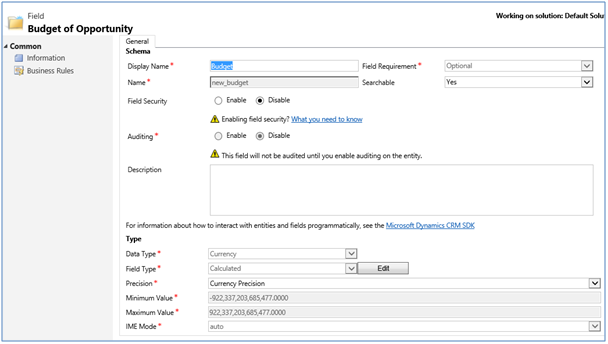2.   To define the calculation for Budget field click on Edit button that appears only when Field type is Calculated or Rollup.This opens a UI similar to Business Rules where we can define calculation.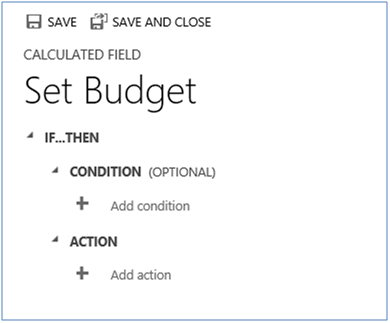1. When setting the action and formula, you get intellisense to support the common functions. Using the intellisense, we can use the related entity by using the lookup fields name of that entity.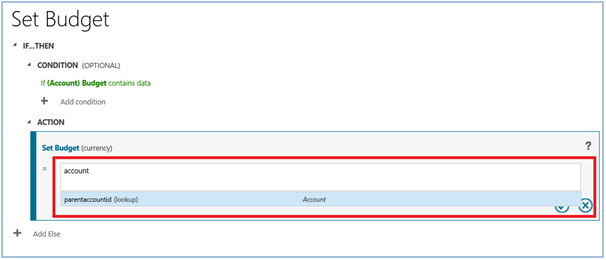2. The lookup fields name allows access to all fields of the look up entity.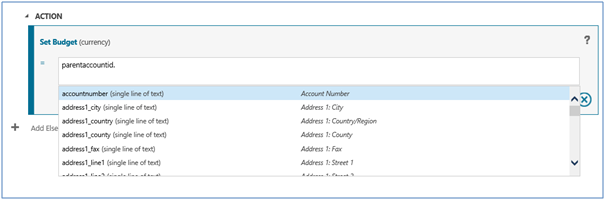3. For above requirement we have defined the conditions and actions as below: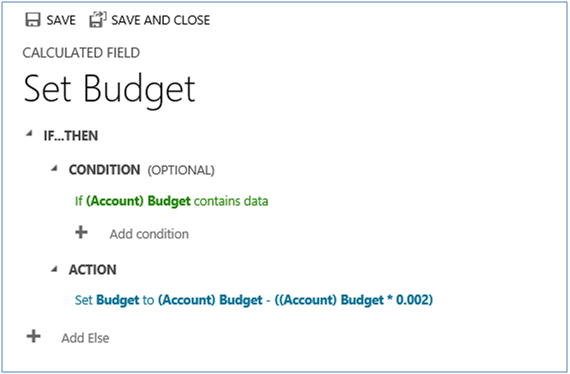Now, when you create a new record, upon Save you will find the Budget automatically set.

Conclusion:

With this extended support we can perform calculations that require related entities fields using calculated fields.

Stuck with migration to Dynamics CRM? Experts at Inogic are always happy to help you, get in touch with us on crm@inogic.com.